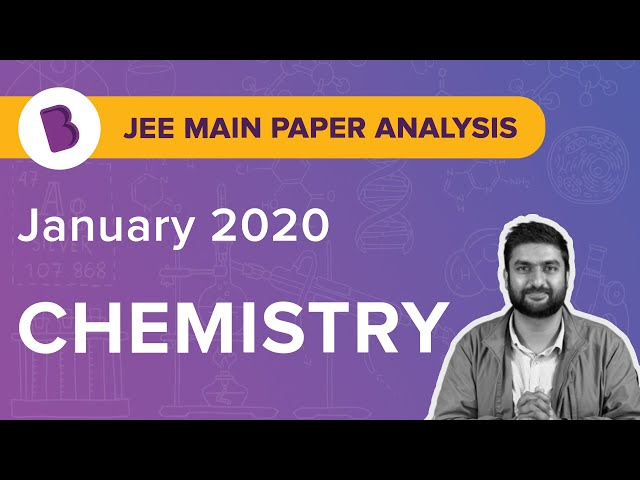Win up to 100% scholarship on Aakash BYJU'S JEE/NEET courses with ABNAT Win up to 100% scholarship on Aakash BYJU'S JEE/NEET courses with ABNAT

# JEE Main 2020 Chemistry Paper With Solutions Jan 7 Shift 1

JEE Main 2020 Paper with Solutions Chemistry – Shift 1 consists of accurate solutions, which helps the students to understand concept clearly. BYJU’S provides clear and step by step solutions prepared by our subject matter experts. Students are recommended to practise these solutions so that they can improve their speed and accuracy. These solutions help to develop confidence among students who are preparing for exams.
January 7 Shift 1 – Chemistry
Question 1. The relative strength of interionic/ intermolecular forces in decreasing order is:
a) ion-dipole > dipole-dipole > ion-ion
b) dipole-dipole > ion-dipole > ion-ion
c) ion-ion > ion-dipole > dipole-dipole
d) ion-dipole > ion-ion > dipoledipole

Ion-ion interactions are stronger because they have stronger electrostatic forces of attraction whereas dipoles have partial charges and hence the electrostatic forces in their case would be relatively weak.

Question 2. Oxidation number of potassium in K2O, K2O2 and KO2, respectively, is :
a) +2, +1, and
$$\begin{array}{l}\frac{+1}{2}\end{array}$$

b) +1, +2 and +4
c) +1, +1 and +1
d) +1, +4 and +2

Alkali metals always possess a +1 oxidation state, whereas oxygen present in K2O (oxide) is -2, and in K2O2 (peroxide) is -1 and in KO2 (superoxide) is

$$\begin{array}{l}\frac{-1}{2}\end{array}$$
.

Question 3. At 350C, the vapour pressure of CS2 is 512 mm Hg and that of acetone is 344 mm Hg. A solution of CS2 in acetone has a total vapour pressure of 600 mm Hg. The false statement amongst the following is :
a) CS2 and acetone are less attracted to each other than to themselves
b) heat must be absorbed in order to produce the solution at 350C
c) Raoult’s law is not obeyed by this system
d) a mixture of 100 mL CS2 and 100 mL acetone has a volume < 200 mL

PTotal = PT = p0A XA+ p0B XB

The maximum value XA can hold is 1, and hence the maximum value of PT should come out to be 512 mm of Hg, which is less than the value of PT observed (600 mm of Hg). Therefore, positive deviation from Raoult’s law that is observed. This implies that A-A interactions and B-B interactions are stronger than A-B interactions. As we know, for a system not obeying Raoult’s law and showing positive deviation, ΔVmix>0, ΔHmix>0 .

Question 4. The atomic radius of Ag is closest to :
a) Ni
b) Cu
c) Au
d) Hg

Because of Lanthanide contraction, an increase in Zeff is observed and so, the size of Au instead of being greater, as is expected, turns out be similar to that of Ag.

Question 5. The dipole moments of CCl4, CHCl3 and CH4 are in the order:
a) CH4 < CCl4 < CHCl3
b) CHCl3 < CH4 = CCl4
c) CH4 = CCl4 < CHCl3
d) CCl4 < CH4 < CHCl3

All the three compounds possess a tetrahedral geometry. In both CCl4 and CH4, µnet= 0, whereas in CHCl3, µnet > 0.

Question 6. In comparison to the zeolite process for the removal of permanent hardness, the synthetic resins method is :
a) less efficient as it exchanges only anions
b) more efficient as it can exchanges only cations
c) less efficient as the resins cannot be regenerated
d) more efficient as it can exchange both cations as well as anions

Question 7. Amongst the following statements, that which was not proposed by Dalton was :
a) matter consists of indivisible atoms
b) when gases combine or reproduced in a chemical reaction they do so in a simple ratio by volume provided all gases are at the same T & P.
c) Chemical reactions involve reorganisation of atoms. These are neither created nor destroyed in a chemical reaction
d) all the atoms of a given element have identical properties including identical mass. Atoms of different elements differ in mass.

When gases combine or react in a chemical reaction they do so in a simple ratio by volume provided all gases are maintained at the same temperature and pressure- Gay-Lussac’s law.

Question 8. The increasing order of pKb for the following compounds will be :

(i) H2N-CH=NH

(ii)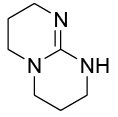(iii) CH3NHCH3

a) ii < iii < i
b) iii < i < ii
c) i < ii < iii
d) ii < i < iii

Weaker the conjugate acid, stronger the base. (ii) is the most basic as it has a guanidine like structure. It has a high tendency of accepting a proton, giving rise to a very stable conjugate acid and hence, is a very strong base. In compound (i), the N is sp2 hybridised and its electronegativity is higher as compared to the compound (iii) which is a 20 amine (sp3 hybridised). So compound (ii) is more basic compared to compound (iii).

So the order of basicity is ii > i > iii and thus the order of pKb value will be iii > i > ii

Question 9. What is the product of the following reaction?

Hex-3-ynal

(i) NaBH4

(ii) PBr3

(iii) Mg/ether

(iv) CO2/H3O+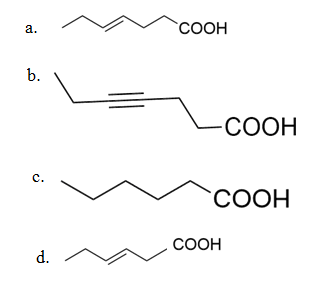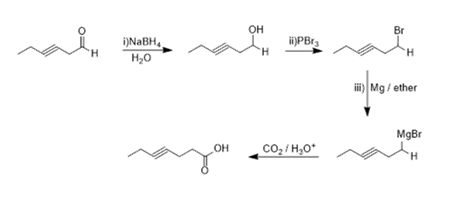Question 10. The number of orbitals associated with quantum number n = 5, ms = +½ is :
a) 11
b) 15
c) 25
d) 50

n = 5; l = (n – 1) = 4; hence the possible sub-shells for n=5 are: 5s, 5p, 5d, 5f and 5g.

The number of orbitals in each would be 1,3,5,7 and 9, respectively and summing them up gives the answer as 25.

Question 11. The purest form of commercial iron is:
a) cast iron
b) wrought iron
c) scrap iron and pig iron
d) pig iron

Question 12. The theory that can completely/ properly explain the nature of bonding in [Ni(CO)4] is:
a) Werner’s theory
b) Crystal Field Theory
c) Molecular Orbital Theory
d) Valence Bond Theory

Question 13. The IUPAC name of the complex [Pt(NH3)2Cl(NH2CH3)]Cl is :
a) Diamminechlorido (methanamine) platinum (II) chloride
b) Bisammine (methanamine) chloridoplatinum (II) chloride
c) Diammine (methanamine) chloridoplatinum (II) chloride
d) Diamminechlorido (aminomethane) platinum (II) chloride

Question 14. 1-methyl ethylene oxide when treated with an excess of HBr produces: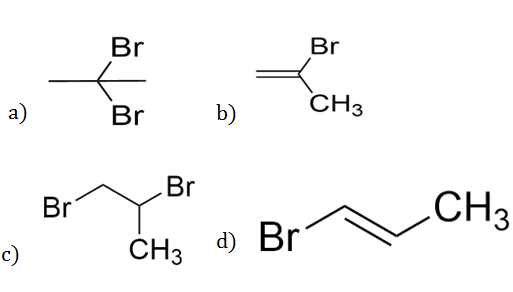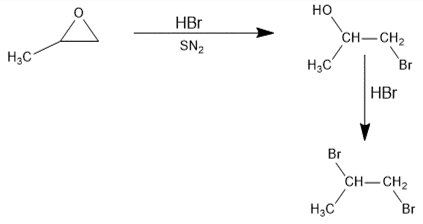Question 15. Consider the following reaction: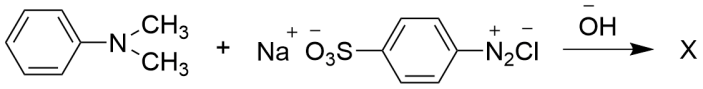The product ‘X’ is used:

a) in protein estimation as an alternative to ninhydrin
c) in laboratory test for phenols
d) in acid-base titration as an indicator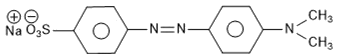X formed is methyl orange.

Question 16. Match the following:

 List I List II i) Riboflavin p) Beri beri ii) Thiamine q) Scurvy iii) Ascorbic acid r) Cheliosis iv) Pyridoxine s) Convulsions
 i ii iii iv a) s q p r b) r p q s c) p r q s d) s r q p

 Vitamins Deficiency diseases i) Riboflavin (Vitamin B2) Cheilosis ii) Thiamine (Vitamin B1) Beri beri iii) Ascorbic acid (Vitamin C) Scurvy iv) Pyridoxine (Vitamin B6) Convulsions

Question 17. Given that the standard potential; (Eo) of Cu2+/Cu and Cu+/Cu are 0.34 V and 0.522 V respectively, the Eo of Cu2+/Cu+ is :
a) +0.158 V
b) -0.158 V
c) 0.182 V
d) -0.182 V

Cu2+ + 2e Cu

E° = 0.340 V

Cu Cu+ + e

E°= -0.522 V

Cu2+ + e ⇾ Cu+

E° = ?

Applying ΔG = nFE° We get,

(-1 × F × E°) = -2 × F × 0.340 + (-1 × F × -0.522)

Solving, we get, E°= 0.158 V

Question 18. A solution of m-chloroaniline, m-chlorophenol and m-chlorobenzoic acid in ethyl acetate was extracted initially with a saturated solution of NaHCO3 to give fraction A. The left over organic phase was extracted with dil. NaOH solution to give fraction B. The final organic layer was labelled as fraction C. Fractions A, B and C, contain respectively:
a) m-chlorobenzoic acid, m-chlorophenol and m-chloroaniline
b) m-chlorophenol, m-chlorobenzoic acid and m-chloroaniline
c) m-chloroaniline, m-chlorobenzoic acid and m-chlorophenol
d) m-chlorobenzoic acid, m-chloroaniline and m-chlorophenol

m-chlorobenzoic acid being the most acidic can be separated by a weak base like NaHCO3 and hence will be labelled fraction A.

m-chlorophenol is not as acidic as m-chlorobenzoic acid, and can be separated by a stronger base like NaOH, and hence can be labelled as fraction B.

m-chloroaniline being a base, does not react with either of the bases and hence would be labelled as fraction C.

Question 19. The electron gain enthalpy (in kJ/mol) of fluorine, chlorine, bromine, and iodine, respectively, are:
a) -333, -325, -349 and -296
b) -333, -349, -325 and -296
c) -296, -325, -333 and -349
d) -349, -333, -325 and -296

Cl > F > Br > I

Question 20. Consider the following reactions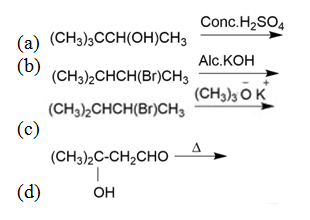Which of these reaction(s) will not produce Saytzeff product?

a) b and d
b) d only
c) a, c and d
d) c only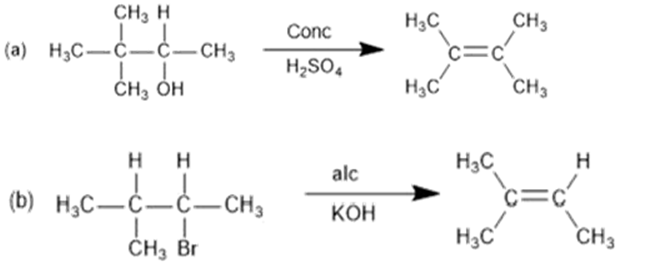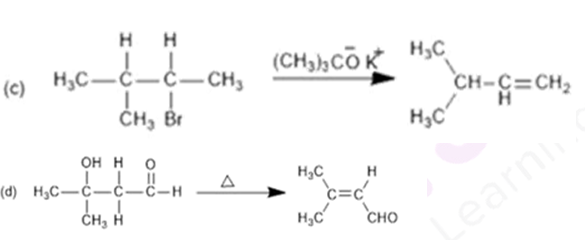Question 21. Two solutions A and B each of 100 L was made by dissolving 4 g of NaOH and 9.8 g of H2SO4 in water, respectively. The pH of the resulting solution obtained from mixing 40 L of Solution A and 10 L of Solution B is:

Molarity of NaOH (4 g in 100 L) = 10-3 M

Molarity of H2SO4 (9.8 g in 100 L) = 10-3 M

Equivalents of NaOH= M × V × nf = 10-3 × 40 × 1= 0.04

Equivalents of H2SO4= M × V × nf = 10-3 × 10 × 2= 0.02

MNaOH . VNaOH . (nf)NaOH – MH2SO4 . VH2SO4. VH2SO4 . (nf)H2SO4 = M. Vtotal

10-3 × 40 × 1 – 10-3 × 10 × 2 = M. 50

M = 4 × 10-4

pOH = -log M

= 4 – 2log2

= 3.4

pH = 14 – 3.4 = 10.6

Question 22. During the nuclear explosion, one of the products is 90Sr with half of 6.93 years. If 1 µg of 90Sr was absorbed in the bones of a newly born baby in place of Ca, how much time, in years, is required to reduce it by 90% if it is not lost metabolically

All nuclear processes follow first order kinetics, and hence

T1/2 = 0.693 / λ

λ = 0.1(year)-1

t = (2.303/ λ) (log (a0)/at)

t = (2.303/ 0.1) (log (a0)/0.1a0)

on solving, t = 23.03 years.

Question 23. Chlorine reacts with hot and concentrated NaOH and produces compounds (X) and (Y). Compound (X) gives white precipitate with silver nitrate solution. The average bond order between Cl and O atoms in (Y) is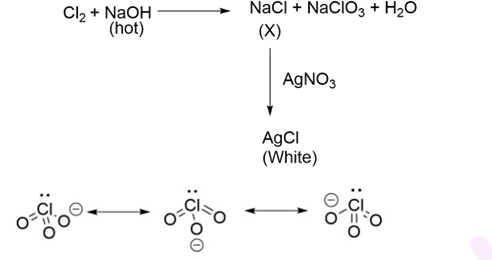Bond order = total no. of bonds / total resonating structures = 5/3 = 1.67.

Question 24. The number of chiral carbons in chloramphenicol is: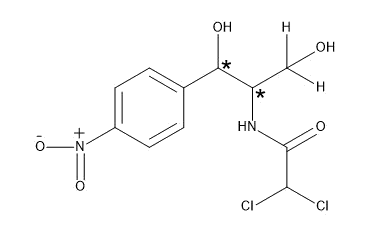Question 25. For the reaction A(l) →2B(g)

∆U= 2.1 kcal, ∆S= 20 calK-1 at 300 K, Hence ∆G in kcal is

∆H= ∆U + ∆ngRT

∆H= 2100 + (2 × 2 × 300) (R=2 calK-1mol-1)

= 3300 cal

∆G= ∆H – T∆S

∆G= 3300 – (300 × 20) = -2.7 kcal.